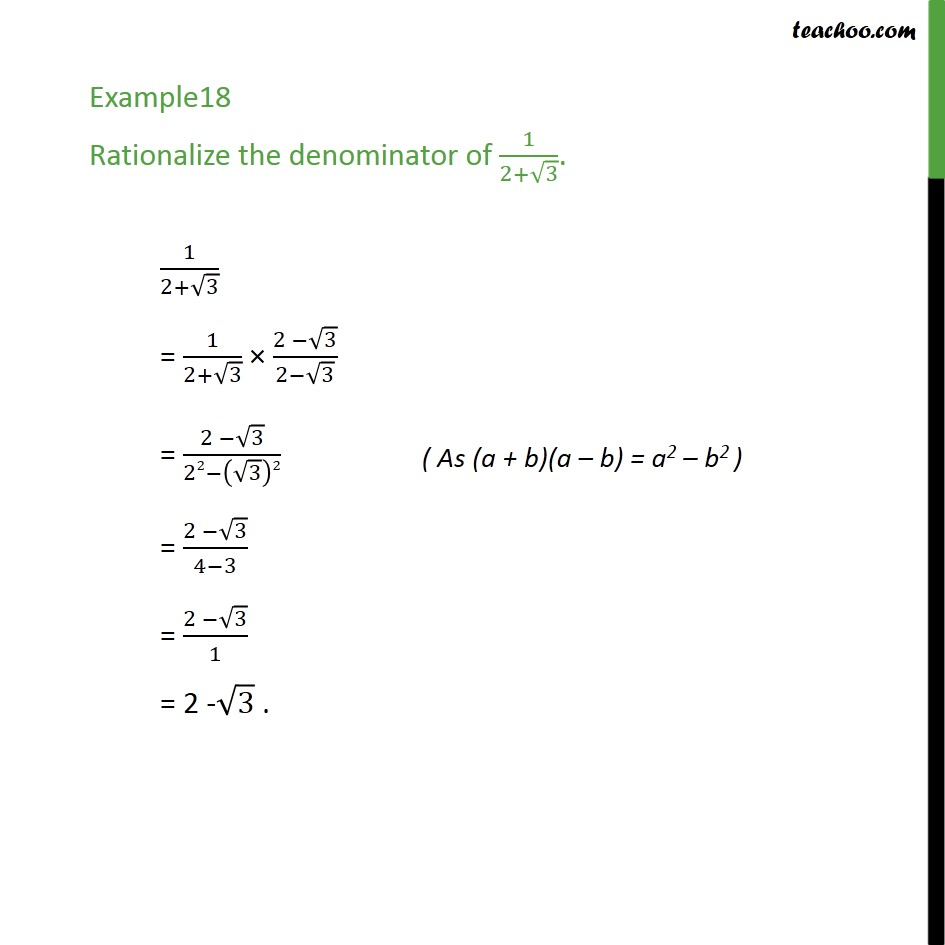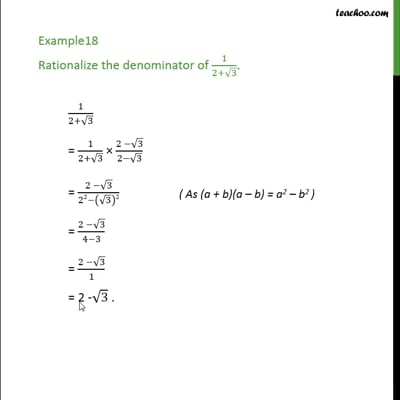Examples

Chapter 1 Class 9 Number Systems
Serial order wiseThis video is only available for Teachoo black users

Maths Crash Course - Live lectures + all videos + Real time Doubt solving!

### Transcript

Example18 Rationalize the denominator of 1/(2+√3). To rationalize, We multiply and divide by 2 - root 3 Let's check the video 1/(2+√3) = 1/(2+√3) × (2 −√3)/(2−√3) = (2 −√3)/(22−(√3)2) = (2 −√3)/(4−3) = (2 −√3)/1 = 2 -√3 .